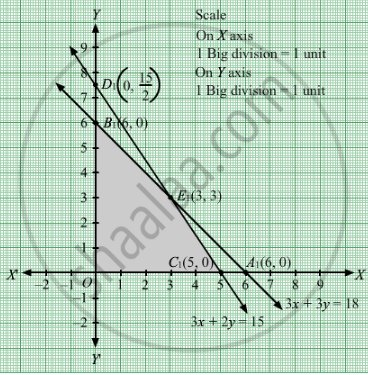# A Manufacturer Produces Two Types of Steel Trunks. He Has Two Machines a and B. How Many Trunks of Each Type Must He Make Each Day to Make Maximum Profit? - Mathematics

Sum

A manufacturer produces two types of steel trunks. He has two machines A and B. For completing, the first types of the trunk requires 3 hours on machine A and 3 hours on machine B, whereas the second type of the trunk requires 3 hours on machine A and 2 hours on machine B. Machines A and B can work at most for 18 hours and 15 hours per day respectively. He earns a profit of Rs 30 and Rs 25 per trunk of the first type and the second type respectively. How many trunks of each type must he make each day to make maximum profit?

#### Solution

Let x trunks of first type and y trunks of second type were manufactured.
Number of trunks cannot be negative.Therefore,

$x, y \geq 0$

According to question, the given information can be tabulated as

 Machine A(hrs) Machine B(hrs) First type(x) 3 3 Second type(y) 3 2 Availability 18 15

Therefore, the constraints are

$3x + 3y \leq 18$

$3x + 2y \leq 15$

He earns a profit of Rs 30 and Rs 25 per trunk of the first type and the second type respectively.Therefore, profit gained by him from x trunks of first type and y trunks of second type is Rs 30x and Rs 25y respectively.
Total profit = Z = $30x + 25y$

which is to be maximised
Thus, the mathematical formulat​ion of the given linear programmimg problem is
Max Z =  $30x + 25y$

subject to

$3x + 3y \leq 18$
$3x + 2y \leq 15$

$x, y \geq 0$

First we will convert inequations into equations as follows:
3x + 3y = 18, 3x + 2y = 15, x = 0 and y = 0

Region represented by 3x + 3y ≤ 18:
The line 3x + 3y = 18 meets the coordinate axes at A1(6, 0) and B1(0, 6) respectively. By joining these points we obtain the line 3x + 3y = 18. Clearly (0,0) satisfies the 3x + 3y = 18. So, the region which contains the origin represents the solution set of the inequation 3x + 3y ≤ 18.

Region represented by 3x + 2y ≤ 15:
The line 3x + 2y = 15 meets the coordinate axes at C1(5, 0) and $D_1 \left( 0, \frac{15}{2} \right)$  respectively. By joining these points we obtain the line 3x + 2y = 15. Clearly (0,0) satisfies the inequation 3x + 2y ≤ 15. So,the region which contains the origin represents the solution set of the inequation 3x + 2y ≤ 15.

Region represented by ≥ 0 and y ≥ 0:
Since, every point in the first quadrant satisfies these inequations. So, the first quadrant is the region represented by the inequations x ≥ 0, and y ≥ 0.

The feasible region determined by the system of constraints 3x + 3y ≤ 18, 3x + 2y ≤ 15, x ≥ 0 and y ≥ 0 are as follows.The corner points are O(0, 0), B1(0, 6), E1(3, 3) and C1(5, 0).

The values of Z at these corner points are as follows

 Corner point Z = 30x + 25y O 0 B1 150 E1 165 C1 150

The maximum value of Z is 165 which is attained at E1(3, 3).
Thus, the maximum profit is Rs 165 obtained when 3 units of each type of trunk is manufactured.

Concept: Graphical Method of Solving Linear Programming Problems
Is there an error in this question or solution?

#### APPEARS IN

RD Sharma Class 12 Maths
Chapter 30 Linear programming
Exercise 30.4 | Q 20 | Page 53